# Step-by-step

Note: some charting functions in the support file may require using an optional free Excel add-in for best performance.

Detailed step-by-step workings of the examples provided below and also available in the illustrative support file you can freely download.

Let us assume a Normal probability distribution, with mean = 50 and standard deviation = 10. We will have 100 units of product in our warehouse, so to limit the size of the simulation.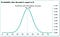Normal probability density function for mean = 50 and stdev = 10. Image source: author from Evo Pricing (CC with attribution)

In a normal distribution, the mean is the same as the mode and the average, and the curve is perfectly symmetrical, so everything is easier. The same logic would nevertheless apply to any other type of probability function.

The most likely outcome is undoubtedly N=50, even if its actual % likelihood is just around 4%. In cumulative terms, the probability of selling up to N pieces grows monotonically from 0 to infinity by definition.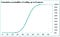Cumulative demand function. Image source: author from Evo Pricing (CC with attribution)

Here we are mixing demand with sales, which may generate some confusion. The cumulative distribution is technically the cumulative demand observed for up to N pieces. A more logical curve is 1 minus the cumulative demand, which is the complement of the above: the probability of demand being at least N.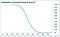Probability of demand being at least N. Image source: author from Evo Pricing (CC with attribution)

Now, for very low numbers of N the probability of selling is close to 100%, as we expect to have demand for N=50 units.

As we approach 50, the S-shaped curve veers progressively towards 0.

In terms of shipment, every shipment cost us 10 and IF we sell one unit, we gain a profit of 60 (100 list price minus 40 for direct product cost). Which means we NET a profit of close to 50 for the first unit shipped, which is 60 minus the all-inclusive cost of shipment of 10.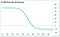Net profit from the shipment on the N-th piece. Image source: author from Evo Pricing (CC with attribution)

By looking at this curve, any shipment generating a marginal profit >0 is economically desirable.

In order to find the optimal shipment, we chart the total profit with N shipments, computed as:

% cumulative profit (minus) N (times) unit cost of shipment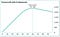Total profit with N shipments. Image source: author from Evo Pricing (CC with attribution)

On this curve we can compute the optimal shipment using standard profit maximization techniques.

Happy rapid responses!

The learnings and benefits of the ‘science of billions’: what if you could access more data than Amazon? Free Evo University project: https://evo.ltd/join

## More from Fabrizio Fantini

The learnings and benefits of the ‘science of billions’: what if you could access more data than Amazon? Free Evo University project: https://evo.ltd/join